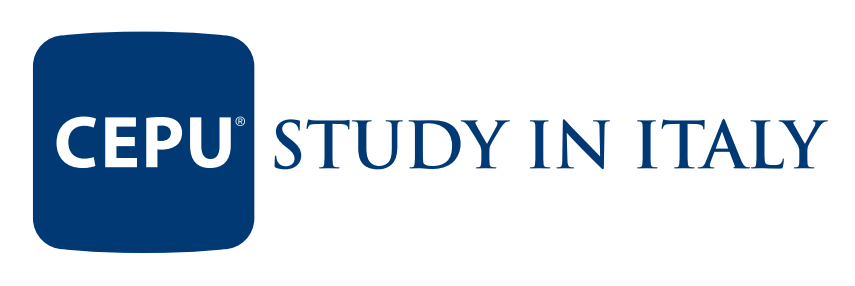意大利CEPU高中已有 名 同学录取本校

•学校所在城市：米兰
•优势专业：

B1

29000欧

• 申请难度

1.专业的艺术课程学习（音乐高中，美术高中）

2.意大利高中文凭可以进入意大利所有大学以后欧盟所有大学

3.更早的取得长期居留

CEPU高中的学校服务:

1.辅导学生功课.

2..生活导师.

CEPU的学费

1.单人间酒店式管理住宿

2校园内网费

3水电暖费，周一至周五清洁费

4高中学费，注册费

5一日三餐

6校车使用费

7监护人费用

6家教辅导课程费用

7意大利语言培训费用

• 意大利语言文化
• 拉丁语言文化
• 希腊语言文化
• 外国语言
• 历史
• 历史和地理
• 哲学
• 数学*
• 物理
• 自然科学**
• 艺术史
• 运动科学
• 天主教活动

• 意大利语言文学
• 拉丁语言文学
• 外国语言文学
• 历史和地理
• 历史
• 哲学
• 数学*
• 物理
• 自然科学**
• 设计和艺术史
• 运动科学
• 天主教教学

• 意大利语言文学
• 外国语言文学
• 历史地理
• 历史
• 哲学
• 数学*
• 物理
• 自然科学**
• 化学***
• 艺术史
• 绘图绘画
• 几何学
• 塑料雕刻
• 艺术实验****
• 机动运动科学
• 天主教教义和相关活动
• 建筑实验
• 建筑环境设计学科

• 意大利语言文学
• 外国语言文学
• 历史地理
• 历史
• 哲学
• 数学*
• 物理
• 自然科学**
• 化学***
• 艺术史
• 图形绘画
• 几何学
• 塑料雕塑
• 艺术实验****
• 机动运动科学
• 天主教教义和相关活动
• 塑形实验室
• 绘画实验室

• 意大利语言文学
• 外国语言文学
• 历史地理
• 历史
• 哲学
• 数学*
• 物理
• 自然科学**
• 化学***
• 艺术史
• 图形绘画
• 几何学
• 塑料雕塑
• 艺术实验****
• 机动运动科学
• 天主教教义和相关活动
• 舞台布景实验室
• 科学几何学技术
• 科学设计技术

• 意大利语言文学
• 外国语言文学
• 历史地理
• 历史
• 哲学
• 数学*
• 物理
• 自然科学**
• 化学***
• 艺术史
• 图形绘画
• 几何学
• 塑料雕塑
• 艺术实验室****
• 机动运动科学
• 天主教教义和相关活动
• 实验室
• 射击课

• 意大利语言文学
• 外国语言文学
• 历史和地理
• 历史
• 哲学
• 数学
• 计算机
• 物理
• 自然科学*
• 设计和艺术史
• 运动科学
• 天主教教学

• 意大利语言文学
• 外国语言文学
• 历史和地理
• 历史
• 哲学
• 数学
• 物理
• 自然科学*
• 经济法
• 运动科学
• 运动学科
• 天主教教学

• 意大利语言文学
• 拉丁语
• 1 第一外语*
• 2 第二外语*
• 3 第三外语*
• 历史和地理
• 历史
• 哲学
• 艺术史
• 数学**
• 物理
• 自然科学***
• 运动科学
• 天主教教学

• 意大利语言文学
• 拉丁语言文学
• 历史和地理
• 历史
• 哲学
• 人文科学*
• 经济法律
• 外国语言文学
• 数学**
• 物理
• 自然科学***
• 艺术历史
• 运动
• 天主教教学

• 意大利语言文学
• 1 外国语言文化
• 2 第二外国语言文化
• 历史和地理
• 历史
• 经济学和法学
• 哲学
• 人文科学*
• 数学**
• 物理
• 自然科学***
• 艺术历史
• 运动科学
• 天主教教学

• 意大利语言文学
• 英语
• 历史
• 数学
• 经济和法律
• 土地和有机学科学
• 运动科学
• 天主教教学
• 物理学
• 化学
• 地理学
• 信息学
• 第二外语
• 企业法
• 信息
• 第二语言
• 企业法
• 法学
• 政治经济学

• 意大利语言文学
• 英语
• 历史
• 数学
• 经济和法律
• 土地和有机学科学
• 运动科学
• 天主教教学
• 物理
• 化学
• 地理
• 企业经济
• 第二语言
• 第三语言
• 旅游企业经济
• 旅游地理
• 旅游法
• 旅游法
• 地域和艺术

• 意大利语言文学
• 英语
• 历史
• 数学
• 法律和经济
• 土地和有机学科学
• 运动科学
• 天主教教学
• 物理学
• 化学
• 绘图技术
• 信息学
• 应用技术
• 数学
• 环境工作保险
• 第二语言
• 企业法
• 法学

• 意大利语言文学
• 英语
• 历史
• 数学
• 法律和经济
• 土地科学和有机科学
• 运动科学
• 天主教教学
• 物理学
• 化学
• 绘图技术
• 信息技术
• 应用科技
• 数学
• 网络技术
• 信息通讯设计技术
• 项目设计和企业管理
• 信息学
• 通讯学法学

• 意大利语言文学
• 历史
• 英语
• 法律和经济
• 数学
• 土地科学和有机科学
• 地理
• 运动科学
• 天主教教学
• 物理
• 化学
• 绘图技巧学
• 信息技术
• 应用科学技术
• 数学
• 动物生产
• 植物生产
• 产品转换
• 农村工程
• 经济，估价，市场和法律
• 土地环境管理
• 生态农业

研究生入学要求：0102030408070605

• --

• --

意大利留学院校评估

（请仔细填写以下信息，我们会在24小时内和你取得联系）插上语言的翅膀，带你飞向梦想

Le lingue ti mettono ali che ti fanno volare verso i tuoi sogni

close
Top